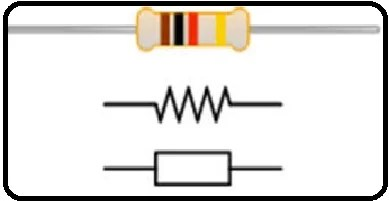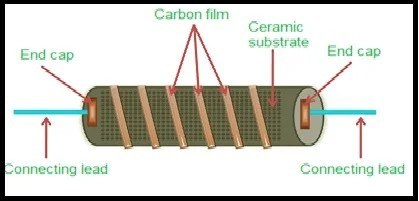# Introduction to Resistors and types of resistors

What are Resistors and their History? Resistor is a passive electronic and electrical component that presents the electrical resistance in a circuit as a circuit element. It consists of two terminals and is used to reduce the current flow, to divide the voltages and to adjust the signal level. It is also used in high power circuits where it reduces the power in the form of heat dissipate and as a load to test the power generators. It works on the principle of ohm’s law. Ohm’s law states that the voltage is directly proportional to circuit resistance and the circuit current which flows in a circuit. The main working principle of every resistor is same but only difference is in their current, voltage, power and the type of material with which it is made. Its ISI unit is ohm. It is available in market from micro ohm of mega ohm. Its resistance is increased or add up when two or more same values of resistors are connected in series, but its current would be same which passed though all resistors as well as voltages are dived across each resistor.

Similarly, when two or more same values of resistors are connected in parallel then its overall resistance is decreased but voltages across each resistor would be same as well the current is divided in each resistor. First time, the resistor was invented by Georg Simon Ohm in 1827 in University of Erlangen. He was the mathematician and he published so many research papers on conduction of heat in molded circuits and he also presented the ohm law. After this, so many resistors are developed on the biases of their manufacturing material. Currently we have been using so many resistors in electronic and electrical systems and we shell discuss them in detail in below paragraphs. A simple resistor with its electrical symbol is shown in figure 1Figure 1 Resistor with its Electrical Symbol

### Types of Resistors

Different types of resistors are available in market for using in electronic and electrical systems. These resistors have different properties based on their construction and manufacturing system and these properties make them to use of different resistor for different applications. Typically, all these resistors are categorized into two types first one is linear and second one is non-linear resistor, but these are further categorized into different types.

Linear Resistors:  Linear resistors are the resistors whose values change with temperature and applied voltages. In other words, in these types of resistors current is directly proportional to applied voltages. Normally two types of resistor have linear properties first one is fixed resistor and second one is variable resistor, but these are further dived into different types.

Fixed Resistor: Fixed resistors are the resistor whose values are fixed mean its values could not change.

Types of Fixed Resistor: Fixed resistors are divided into four types,

Wire Wound Resistors: Wire wound resistor is a type of resistor which is made up with insulating rod or core, at which resistor wire is wrapped around this rod or core. Resistor wire is generally made up with magnetism or tungsten or nickel material and core is made up with press bound paper or ceramic clay material or beaklike etc. If we talk about the cost of wire, then magnetism wire wound resistors are so much costly and these are mainly used in sensitive test equipment such as in wheat stone bridge etc. Their power rating which is easily available in market is 2 watts to up to 100 watts, ohmic resistance 1ohm 200 K ohm and these could be operated safely at 350 Co temperature. A simple wire wound resistor is shown in figure 2Figure 2 Simple Wire Wound Resistor

Carbon Composition Resistors: These types of resistors are made out with the mixture of graphite or powdered carbon or resin binder or insulation filler. The ratio of insulating material in these resistors decided the value of resistor. These are made up in rod shapes and two metal caps are connected at the both ends of this rod which are easily sold in PCB. They are very reliable, very cheap and small in size therefore they occupy less space. They are available in market in different ohms and power rating. A simple carbon composition resistor is shown in figure 3Figure 3 Simple Carbon Composition Resistor

Thin Film Resistor: Typically, thin film resistors are made up with a resistive material of high grid ceramic rod. On this insulating rod which are normally available in market in the shape of glass or tube, a thin layer of conductive material is layer and overlaid to form a thin film resistor. This resistor is further divided into two types first one is carbon film resistor and second one is metal film resistor.

Carbon Film Resistor: Carbon film resistors consist of a high grid ceramic material rod that is called substrate, and, on this substrate, a very thin resistive layer of carbon or film is layer and overlaid to form a carbon film resistor. Because they have negligible resistance, high stability and wide operating range as compared to other types of resistors therefore they are mainly used in electronic circuits

Metal Film Resistor: The construction of metal film resistor is almost same as carbon film resistor but the only difference between them is that its core is made up with metal material instead of carbon. They are very cheap, tiny and reliable in operation. Its temperature co-efficient is very low almost + 2 pp/mC0. These are used in that places where low noise and stability is not so much important. A metal film resistor is shown in figure 5Figure 5 Metal Film Resistor

Thick Film Resistor: The manufacturing method of thick film resistor is same as metal film resistor or thin film resistor, but the difference is only that it has made up with thick layer of resistive material instead of thin layer. Therefore, it is called thick film resistor.

Variable Resistor: Variable resistors are the resistor whose values changed by moving the knob. These are further divide into three types.

Potentiometers: It is the type of resistor whose resistance value is changed by rotating the wiper which is connected to control shaft. It consists of three terminals, resistance between external terminal is fixed and resistance between one external and one central terminal is variable. These are used for controlling the voltages of electronic circuit.

Rheostats: Rheostat is a device which consists of three terminals and is used for limiting the current manually or by hand. These are also known as wire wound variable resistors or tap changing resistors. These are available in market in different construction but the purposes of all these are same.

Trimmer Resistors: Trimmer is a type of potentiometer, which consists on an addition screw, whose position is changed with the help of screw driver. By changing the position of this screw, the value of resistance is changed.